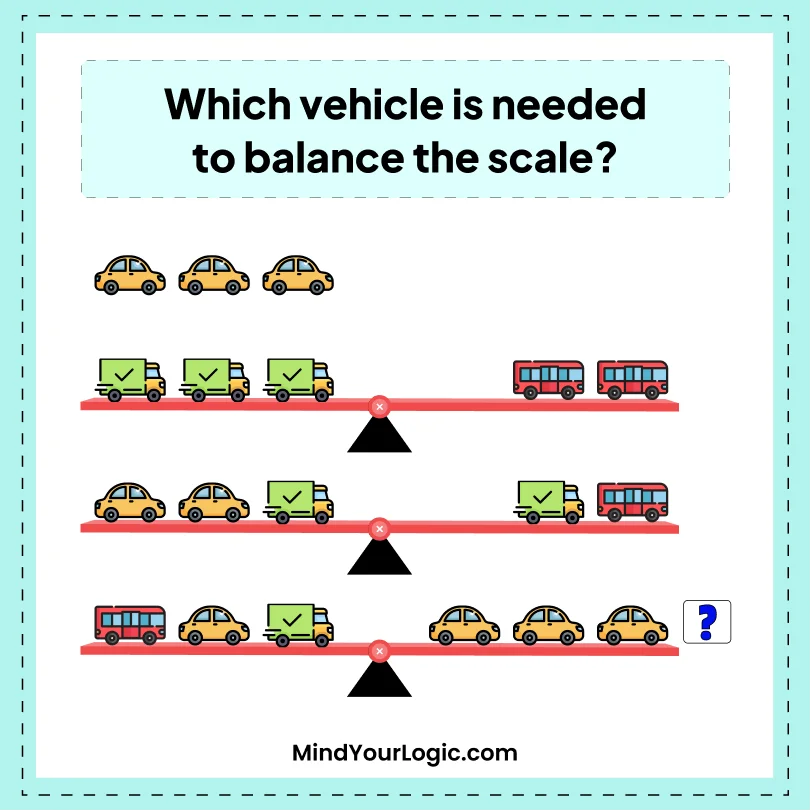# Vehicle make scale balance - Math Riddle

###### 112.Math Riddles
`Which vehicle is needed to balance the scale?`• A.Carriage
• B.Bus
• C.Car
• D.none of them
• ```Explanation :

Let us assume
Carriage = 2, the Car = 3 and the Bus = 6
6+3+2= 3+3+3
If we remove carriage it will be balancedCarriage = 2, the Car = 3 and the Bus = 6
6+3+2= 3+3+3
If we remove carriage it will be balanced```